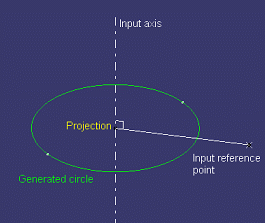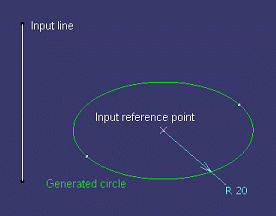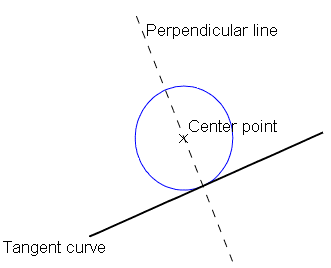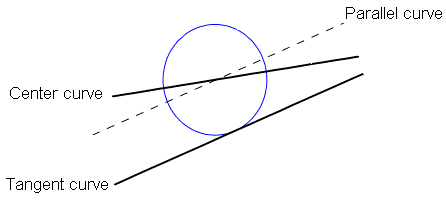# Circle Constructors

 circleCtrRadius (center: Point, support: Surface, radius: Length, limits: Integer,  start: Angle, end: Angle): Circle Creates a circular arc from its center and radius. If the argument 4 is 0, arguments 5 and 6 are taken into account. Otherwise, a circle is created. circleCtrPt (center: Point, point: Point, support: Surface, limits: Integer, start: Angle, end: Angle): Circle Creates a circular arc from its center and another point located on the circle.  If the argument 4 is 0, arguments 5 and 6 are taken into account. Otherwise, a circle is created. circle2PtsRadius (point1: Point, point2: Point, support: Surface, radius: Length, orientation: Boolean, limits: Integer): Circle   Creates a circular arc. The points specified in arguments 1 and  2 are located on the arc to be created and define the arc limits when the integer specified in the argument 6 is 0. When 0 is specified in the argument 6, modifying the argument 5 boolean value allows you to display the alternative arc. Circle3Pts (point1: Point, point2: Point, point3: Point, limits: Integer): Circle Creates one or more circular arcs passing through three points. When 0 is specified in the argument 4, the first and third points define the arc limits. When 1 is specified in the argument 4 the whole circle is defined. When 2 is specifies in the argument 4 the direct circle is defined. When 3 is specified in the argument 4, the complementary circle is defined. circleBitgtRadius (curve1: Curve, curve2: Curve, support: Surface, radius: Length, orientation1: Boolean, orientation2: Boolean, limits: Integer): Circle Creates one or more circular arcs tangent to two curves. When 0 is specified in the argument 7, the tangency points define the arc limits. Modifying orientation1 argument value allows you to reverse the arc orientation with respect to the curve1 curve (there may be no solution). Modifying orientation2 argument value allows you to reverse the arc orientation with respect to the curve2 curve. circleBitgtradius (circleBitgtRadius curve: Curve, point: Point, support; Surface, radius: Length, orientation1: Boolean, orientation2: Boolean, limits: Integer): Circle Creates one or more circular arcs tangent to two curves. circleBitgtPoint (curve1:Curve, curve2: Curve, pt: Point, support: Surface, orientation1: Boolean, orientation2: Boolean, limits: Integer) : Circle Creates one or more circular arcs tangent to two curves and passing through a point on the second curve. When 0 is specified in the argument 7, the tangency points define the arc limits. Modifying the orientation1 argument value allows you to reverse the arc orientation with respect to the crv1 curve  (there may be no solution). Modifying the orientation2 argument value allows you to reverse the arc orientation with respect to the crv2 curve. circleTritgt (curve1: Curve, curve2: Curve, curve3: Curve, support: Surface, orientation1: Boolean, orientation2: Boolean, orientation3: Boolean, limits: Integer): Circle Creates one or more circular arcs tangent to three curves. When 0 is specified in the argument 8, the tangency points define the arc limits. Modifying the value of an orientation argument allows you to reverse the arc orientation with respect to the curve which has the same order in the argument  specification (orientation1 to be associated with curve1). circleCtrAxis (axis: Line, point: Point, radius: Length, start: Angle, end: Angle, projectionmode: Boolean, limits: Integer): Circle Creates a circle using a point and axis/line as input elements and corresponds to `Center and axis` type of circle in the user interface. axis: Circle created on a plane perpendicular to this axis. point: Element used for center computation. radius: Radius of the circle created. start: Start angle of circle. Used only if limits value is 0. end: End angle of circle. Used only if limits value is 0. projectionmode: ProjectionMode = True implies that the point specified will be projected on to axis/line and will be used as center of the circle, projectionMode = False implies that point will be center of the circle. limits: Circle limitation type. 0 for Angles i.e Part arc, 1 for whole circle"Point and axis" with projection "Point and axis" without projection circleCtrtgt (point: Point, curve: Curve, curve orientation: Boolean, tangent orientation: Boolean, support: Surface): Circle Creates a circle using center, curve and support and corresponds to `Center and tangent` type of circle in the user interface. Orientations are needed to select the proper circle in case of multiple solutions. point: center of the circle curve: curve to which the created circle will be tangent. curve orientation: Tangent curve orientation for circle computation. tangent orientation: Tangent orientation of tangent curve for circle computation. support: support surface.circleCtrtgt (curve1: Curve, curve2: Curve, curve2 orientation: Boolean, curve2 tgt orientation: Boolean, support: Surface, radius: Length, curve1 orientation: Boolean, curve1 tgt orientation: Boolean): Circle This is used to create a circle using center, curve and support and corresponds to `Center and tangent` type of circle in the user interface. Orientations are needed to select the proper circle in case of multiple solutions. curve1: Center element on which the center of circle lies. It is a curve curve2: Curve to which the created circle will be tangent. curve2 orientation: Tangent curve orientation for circle computation. curve2 tgt orientation: Tangent orientation of tangent curve for circle computation. support: support surface. radius: Radius of the circle created curve1 orientation: Center element's orientation for circle computation. curve1 tgt orientation: Tangent orientation of center element for circle computation.